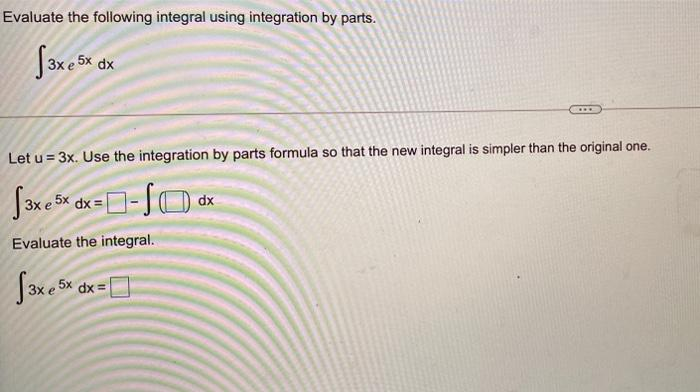# Question Evaluate the following integral using integration by parts. ( 3x e 5x dx Let u = 3x. Use the integration by parts formula so that the new integral is simpler than the original one. [3x e5x dx = D-SO. Зх. dx Evaluate the integral. (3xesx dx =